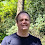### Java Puzzle operator confusion

Why does this
```int _=1, __=2;
int j = __ ^_^ __, k = __-- -+- _ -+- --__;
System.out.println(j + " " + k);
```
print the following?
```1 3
```

1.This comment has been removed by the author.

2.right expressions in Java are evaluated from left to right (my mistake) so let's think like Java now:

i = 2
j = 1
i-- + j = 3
i-- + j + --i = 3 (i is already evaluated and equals 1 so --i = 0)

right?

3.Brillant, you made it look easy. :)

4.10
^01
^10
=
01
2 + 1 + 0 = 3

5.to compare:
Java 1 3
C/C++ 1 4
Python 1 5
Perl 1 3

6.@Przemyslaw, Thank you for the comparison. ;)